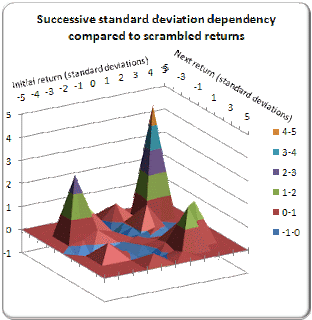### Fake data, part 4: Perturbing volatility

And here is part 4, which I drafted years ago but never published on my blog until now. I didn't quite get done with this project the way I wanted, but it's been a long time and I've moved on.

Now I want to see if there is any dependency between successive moves in a market, compared to a random walk.

I first built a 21×21 table (corresponding to the integers -10 to +10 on each axis) where each cell contains a count of how many times a move of [row] standard deviations was followed by a move of [column] standard deviations. For example, if the cell at [-2,1] contains the value 87, that means during the entire history of the market, a return of -2 standard deviations was followed by a return of +1 standard deviations 87 times. I used 48 years of data, about 12,000 values for the S&P 500 index.

Then I performed a Monte Carlo test. I scrambled the order of log returns (not prices) several hundred times and constructed a similar table containing the average count of each time a move of one size followed a move of another size.

I then made a third table as a ratio of the original table and the Monte Carlo table, ignoring any cells having a statistically insignificant count less than 6.

If the S&P 500 behaves randomly, no pattern should have emerged. But something did emerge. The following two pictures show graphical representations of my third table, in contour and 3D formats:This is interesting. There's a definite peak in the four corners at ±3 standard deviations. In fact the maximum [-3,3] indicates that a 3-sigma drop followed by a 3-sigma bounce is four times more likely to happen in the market than in a random walk using the same distribution of returns.

There's no telling what happens beyond 3 standard deviations because even with 48 years of data, that still isn't enough to get a good sampling of the outliers.  Slightly negative regions (in blue) appear closer to the zero-sigma axes, indicating that sideways moves are less likely to follow non-sideways moves, and vice versa, than a random walk would suggest.

I see a slight ridge connecting the two peaks along the x=y axes (which slants from the top left to lower right of the contour plot). This indicates a very slight directional dependency, meaning that a move of some magnitude and direction would be followed by a move of the same magnitude and direction, slightly more often than one would observe in a random walk.

Note that these are still low probabilities. The 3-sigma drop-and-bounce occurs less than 1% of the time in randomized data, so if the market does this four times more often (4%), the market will still do something else 96% of the time.

So what does this mean? It might mean that volatility (standard deviation), which is calculated once for the entire history, may oscillate up and down, and the dependency we observe is simply an artifact of volatility being high for a couple of days, then low for some more days, then high, then low, and so on.

OK, let's see what happens in our black swan distribution if we perturb volatility σ so that it wanders around its intended value. We could simply add a small-amplitude periodicity to σ, but that wouldn't be realistic. If one plots σ calculated over some finite interval for any financial market, it appears to oscillate around a value randomly.

What we need is a random oscillator that we can control. We need a random walk that doesn't wander aimlessly all over, but rather is attracted to zero. The random walk oscillator offers a solution.